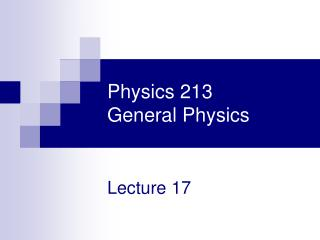# Physics 213 General Physics - PowerPoint PPT PresentationDownload PresentationPhysics 213 General Physics

Physics 213 General Physics
Download Presentation## Physics 213 General Physics

- - - - - - - - - - - - - - - - - - - - - - - - - - - E N D - - - - - - - - - - - - - - - - - - - - - - - - - - -
##### Presentation Transcript

1. 0 Physics 213General Physics Lecture 17

2. Exam 2 Distribution

3. Last Meeting: Mirrors and Lenses Today: Interference

4. Huygen’s Principle • Huygen’s Principle is a geometric construction for determining the position of a new wave at some point based on the knowledge of the wave front that preceded it • All points on a given wave front are taken as point sources for the production of spherical secondary waves, called wavelets, which propagate in the forward direction with speeds characteristic of waves in that medium • After some time has elapsed, the new position of the wave front is the surface tangent to the wavelets Wave fronts

5. Huygen’s Construction for a Plane Wave • At t = 0, the wave front is indicated by the plane AA’ • The points are representative sources for the wavelets • After the wavelets have moved a distance cΔt, a new plane BB’ can be drawn tangent to the wavefronts

6. Huygen’s Construction for a Spherical Wave • The inner arc represents part of the spherical wave • The points are representative points where wavelets are propagated • The new wavefront is tangent at each point to the wavelet

7. Huygen’s Principle and the Law of Reflection • The Law of Reflection can be derived from Huygen’s Principle • AA’ is a wave front of incident light • The reflected wave front is CD

8. Huygen’s Principle and the Law of Reflection, cont • Triangle ADC is congruent to triangle AA’C • θ1 = θ1’ • This is the Law of Reflection

9. Young’s Double Slit Experiment, Diagram • The narrow slits, S1 and S2 act as sources of waves • The waves emerging from the slits originate from the same wave front and therefore are always in phase

10. Resulting Interference Pattern • The light from the two slits form a visible pattern on a screen • The pattern consists of a series of bright and dark parallel bands called fringes • Constructive interference occurs where a bright fringe appears • Destructive interference results in a dark fringe

11. Interference Patterns • Constructive interference occurs at the center point • The two waves travel the same distance • Therefore, they arrive in phase

12. Interference Patterns, 2 • The upper wave has to travel farther than the lower wave • The upper wave travels one wavelength farther • Therefore, the waves arrive in phase • A bright fringe occurs

13. Interference Patterns, 3 • The upper wave travels one-half of a wavelength farther than the lower wave • The trough of the bottom wave overlaps the crest of the upper wave • This is destructive interference • A dark fringe occurs

14. Interference Equations • The path difference, δ, is found from the tan triangle • δ = r2 – r1 = d sin θ • This assumes the paths are parallel • Not exactly parallel, but a very good approximation since L is much greater than d

15. Interference Equations, 2 • For a bright fringe, produced by constructive interference, the path difference must be either zero or some integral multiple of the wavelength • δ = d sin θbright = m λ • m = 0, ±1, ±2, … • m is called the order number • When m = 0, it is the zeroth order maximum • When m = ±1, it is called the first order maximum

16. Interference Equations, 3 • When destructive interference occurs, a dark fringe is observed • This needs a path difference of an odd half wavelength • δ = d sin θdark = (m + ½) λ • m = 0, ±1, ±2, …

17. Interference Equations, 4 • The positions of the fringes can be measured vertically from the zeroth order maximum • y = L tan θ  L sin θ • y=Lmλ/d • Assumptions • L>>d • d>>λ • Approximation • θ is small and therefore the approximation tan θ  sin θ can be used

18. Interference Equations, final • For bright fringes • For dark fringes

19. Phase Changes Due To Reflection • An electromagnetic wave undergoes a phase change of 180° upon reflection from a medium of higher index of refraction than the one in which it was traveling • Analogous to a reflected pulse on a string

20. Phase Changes Due To Reflection, cont • There is no phase change when the wave is reflected from a boundary leading to a medium of lower index of refraction • Analogous to a pulse in a string reflecting from a free support

21. Interference in Thin Films • Ray 1 undergoes a phase change of 180° with respect to the incident ray • Ray 2, which is reflected from the lower surface, undergoes no phase change with respect to the incident wave

22. Interference in Thin Films, Interference Conditions – Normal Incidence • Ray 2 also travels an additional distance of 2t before the waves recombine • For constructive interference • 2 n t = (m + ½ ) λ m = 0, 1, 2 … • This takes into account both the difference in optical path length for the two rays and the 180° phase change • For destruction interference • 2 n t = m λ m = 0, 1, 2 …